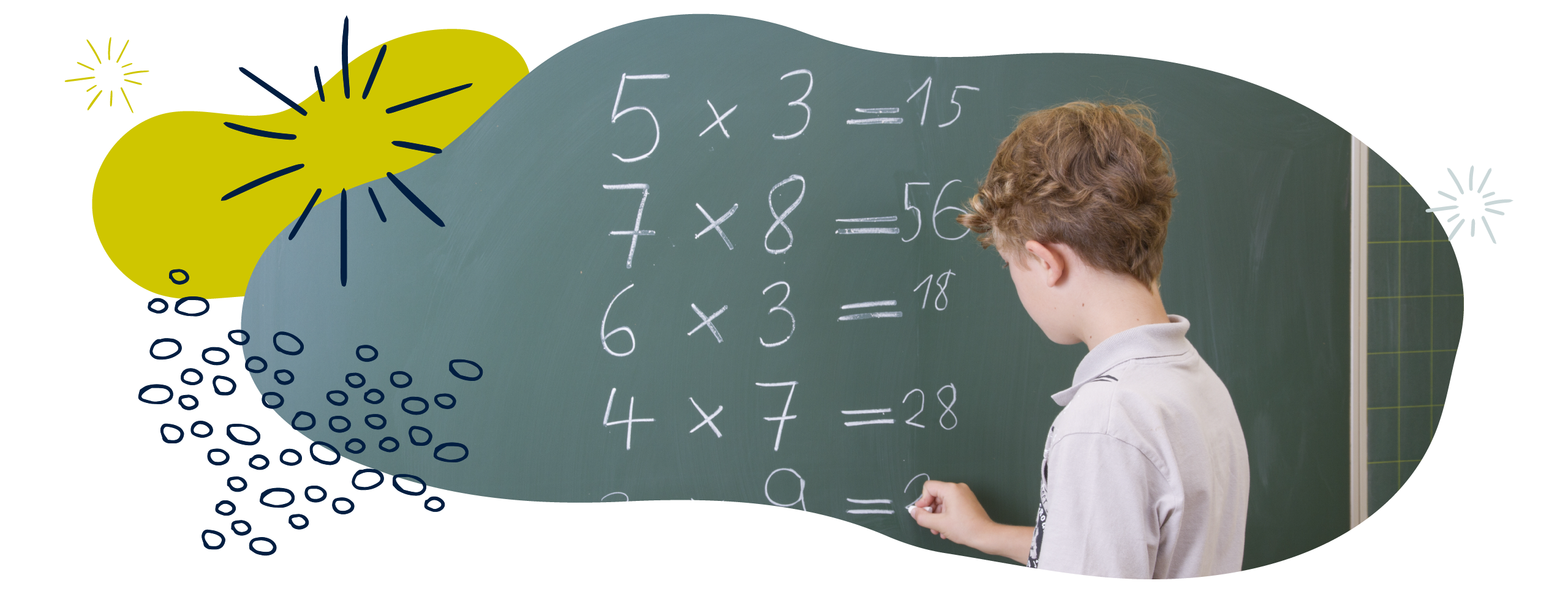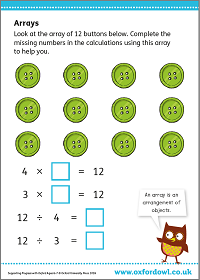We use cookies to enhance your experience on our website. By continuing to use our website, you are agreeing to our use of cookies. You can change your cookie settings at any time. Find out more# Multiplication & division in Year 1 (age 5–6)

In Year 1, your child will learn to read, write, and understand mathematical ideas using multiplication (×), division (÷), and equals (=) signs. They will start to use arrays and will use grouping and sharing to divide.

The key word for this section is array.

## What your child will learn

Take a look at the National Curriculum expectations for multiplication and division in Year 1 (age 5–6):

#### Solve one-step problems involving multiplication and division

Your child will be able to count in steps of 2, 5, and 10. They will start linking this to the idea of multiplication as ‘repeated addition’. For example, they will know that 3 × 2 is the same as 2 + 2 + 2.

Your child will be expected to solve simple division problems through sharing and grouping. For example:

10 ÷ 2 can be thought of as sharing 10 objects into 2 equal groups. This gives us 5 in each group.

10 ÷ 2 can be thought of as finding how many groups of 2 there are in 10. This gives us 5 groups of 2.

Your child will use objects and drawings to help them understand multiplication and division. They will make and use arrays to solve problems. (An array is a set of objects arranged in rows and columns to make a rectangle.)

They will begin to understand the relationship between multiplication and division, and will calculate with concrete objects, pictorial representations and arrays, and the support of their teacher.

## How to help at home

There are lots of ways you can help your child to understand multiplication and division. Here are just a few ideas:

### 1. Practise multiplication

It could help your child to think of multiplication as repeated addition. They should understand that when we multiply, we are adding the same amount each time. For example, 3 × 2 is the same as 2 + 2 + 2.

Using objects might help your child to understand adding objects that are grouped in 2s, 5s, and 10s. There are lots of opportunities to do this using things around your home! You could use pairs of shoes or socks to practise counting in twos, gloves to count in fives, and ice cube trays to practise counting in tens. Help your child to draw pictures of the items they have counted and use these to practise counting in steps of 2, 5, or 10 at any time.

Your child will use arrays to help them with multiplication. Arrays are sets of objects arranged in rows and columns to make a rectangle. For example, egg boxes, muffin trays, ice cube trays, and chocolate bars with rows of pieces are all arrays.

Baking is a great way to use arrays. For example, explain to your child that you need to make 12 muffins. Look at the baking tray. There are 3 rows and 4 columns. Count each column of 4 to show there are 12 holes in total. If we turn the tin around the other way it will be the same.

### Activity: ArraysComplete the calculations using an array.

### 2. Try different methods of division

In Year 1, your child will begin to understand division as sharing and grouping. There are many easy ways you can support this at home.

#### Division by sharing

Ask your child to practise division by sharing objects equally.For example, 8 ÷ 2 could be solved by sharing 8 objects into 2 equal groups. Using objects such as your child’s toys, items of food, or buttons helps them visualise what the calculation means and keeps them interested.

Ask your child to find out how many objects there are to begin with. Can they share the objects equally between the two of you? Help them to share objects one at a time between the two groups. When all the objects have been shared, ask your child how many there are in each group. If you have the same number, explain that your child has shared the objects equally into two groups which is the same as dividing by two.

If you do not have the same number, ask your child if the objects have been shared equally. For example, ‘we started with 11 objects and shared them into two groups and I have 6 objects and you have 5 objects. Are they equal? If they are not equal then 11 cannot be shared equally into two groups so 11 cannot be divided by 2 equally using whole numbers or objects.’

### Video: How to do division by sharing

Homework help! Find out how to do division by sharing.

#### Division by grouping

It’s important that your child also understands division as grouping. Again, you can use any objects from around your home to practise division as grouping.

Ask your child to find out how many there are in a group of objects. Explain to them that you want to find out how many groups of two there are in the total group. Help your child to take two items at a time and count how many groups of two there are in the total number.

For example, if you had 8 objects and wanted to divide by grouping this could be seen as ‘How many groups of 2 are there in 8?’. Ask and support your child to explain that they have divided the 8 objects by grouping them in twos and finding out how many groups of 2 there are in the total group of 8 objects.

### Video: How to do division by grouping

Homework help! Find out how to do division by grouping.

### 3. Use mathematical language

It’s helpful to be able to use the language of multiplication and division when talking with your child, because being able to reason and communicate mathematically is one of the main aims of the National Curriculum. It also makes it much easier for your child to understand calculations when they are learning in school.

Multiplication language includes multiply, times, repeated, addition, equal groups, and arrays. Division language includes divide, sharing equally, grouping equally, and arrays.

You can help your child learn these terms by asking them to use this language to explain how they have solved a problem. It may be helpful to have the mathematical words written on cards and to ask your child to use some of the words in their explanations.